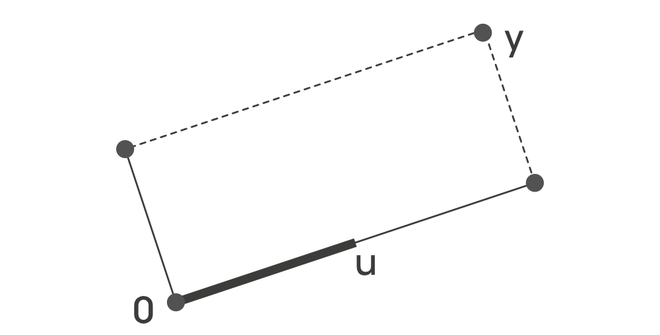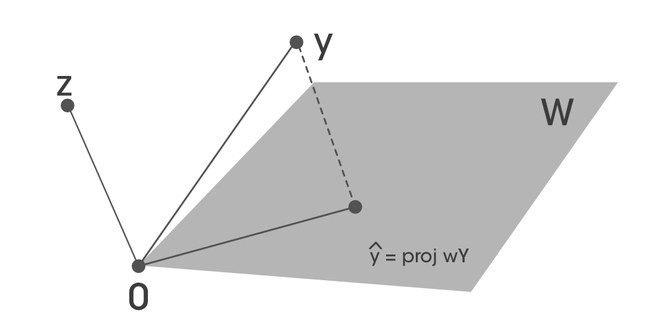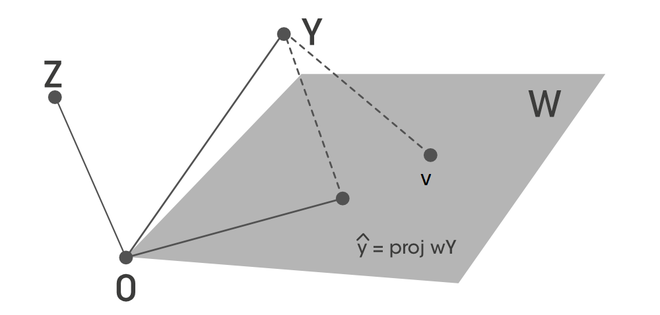GeeksforGeeks App
Open AppBrowser
Continue

# Orthogonal Projections

### Orthogonal Sets:

A set of vectorsinis called orthogonal set, if. if### Orthogonal Basis

An orthogonal basis for a subspace W ofis a basis for W that is also an orthogonal set.

Let S =be the orthogonal basis for a W ofis a basis for W that is also a orthogonal set. We need to calculatesuch that :Let’s take the dot product of u_1 both side.Since, this is orthogonal basis. This gives:We can generalize the above equation### Orthogonal Projections

Suppose {u_1, u_2,… u_n} is an orthogonal basis for W in. For each y in W:Let’s takeis an orthogonal basis forand W = span. Let’s try to write a write y in the formbelongs to W space, and z that is orthogonal to W.whereand[Tex]y= \hat{y} + z[/Tex]

Now, we can see that z is orthogonal to bothandsuch that:#### Orthogonal Decomposition Theorem:

Let W be the subspace of. Then each y incan be uniquely represented in the form:whereis in W and z in W^{\perp}. Ifis an orthogonal basis of W. then,thus:Then,is the orthogonal projection of y in W.#### Best Approximation Theorem

Let W is the subspace of, y any vector in. Let v in W and different from. Thenalso in W.is orthogonal to W, and also orthogonal to.  Then y-v  can be written as:Thus:Thus, this can be written as:and## References:

My Personal Notes arrow_drop_up